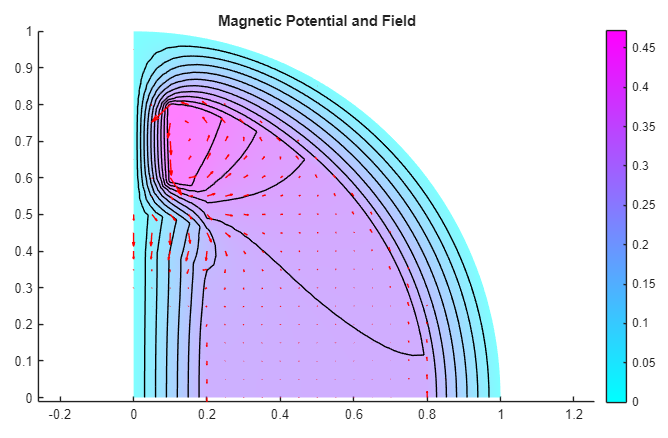## Magnetic Field in Two-Pole Electric Motor

Find the static magnetic field induced by the stator windings in a two-pole electric motor. Assuming that the motor is long and the end effects are negligible, you can use a 2-D model. The geometry consists of three regions:

• Two ferromagnetic pieces: the stator and the rotor, made of transformer steel

• The air gap between the stator and the rotor

• The armature copper coil carrying the DC current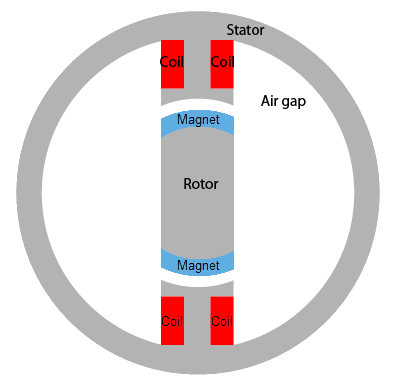The magnetic permeability of air and of copper are both close to the magnetic permeability of a vacuum, μ = μ0. The magnetic permeability of the stator and the rotor is μ = 5000μ0. The current density J is 0 everywhere except in the coil, where it is 10 A/m2.

The geometry of the problem makes the magnetic vector potential A symmetric with respect to the y-axis and antisymmetric with respect to the x-axis. Therefore, you can limit the domain to x ≥ 0, y ≥ 0, with the default boundary condition

`$n\cdot \text{\hspace{0.17em}}\left(\frac{1}{\mu }\nabla A\right)=0$`

on the x-axis and the boundary condition A = 0 on the y-axis. Because the field outside the motor is negligible, you can use the boundary condition A = 0 on the exterior boundary.

First, create the geometry in the PDE Modeler app. The geometry of this electric motor is a union of five circles and two rectangles. To draw the geometry, enter the following commands in the MATLAB® Command Window:

```pdecirc(0,0,1,'C1') pdecirc(0,0,0.8,'C2') pdecirc(0,0,0.6,'C3') pdecirc(0,0,0.5,'C4') pdecirc(0,0,0.4,'C5') pderect([-0.2 0.2 0.2 0.9],'R1') pderect([-0.1 0.1 0.2 0.9],'R2') pderect([0 1 0 1],'SQ1')```

Reduce the geometry to the first quadrant by intersecting it with a square. To do this, enter `(C1+C2+C3+C4+C5+R1+R2)*SQ1` in the Set formula field.

From the PDE Modeler app, export the geometry description matrix, set formula, and name-space matrix to the MATLAB workspace by selecting Export Geometry Description, Set Formula, Labels... from the Draw menu.

In the MATLAB Command Window, use the `decsg` function to decompose the exported geometry into minimal regions. This command creates an `AnalyticGeometry` object `d1`. Plot the geometry `d1`.

```[d1,bt1] = decsg(gd,sf,ns); pdegplot(d1,"EdgeLabels","on","FaceLabels","on")```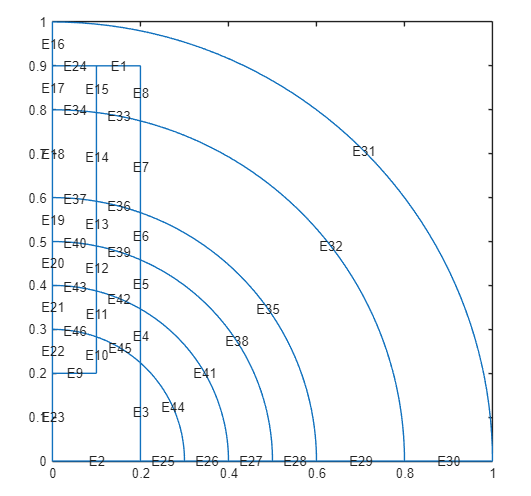Remove unnecessary edges using the `csgdel` function. Specify the edges to delete as a vector of edge IDs. Plot the resulting geometry.

```[d2,bt2] = csgdel(d1,bt1,[1 3 8 25 7 2 12 26 30 33 4 9 34 10 31]); pdegplot(d2,"EdgeLabels","on","FaceLabels","on")```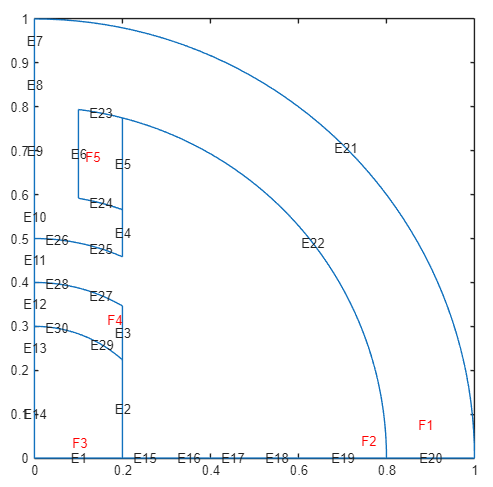Create an electromagnetic model for magnetostatic analysis.

`emagmodel = createpde("electromagnetic","magnetostatic");`

Include the geometry in the model.

`geometryFromEdges(emagmodel,d2);`

Specify the vacuum permeability value in the SI system of units.

`emagmodel.VacuumPermeability = 1.2566370614E-6;`

Specify the relative permeability of the air gap and copper coil, which correspond to the faces 3 and 4 of the geometry.

```electromagneticProperties(emagmodel,"RelativePermeability",1, ... "Face",[3 4]);```

Specify the relative permeability of the stator and the rotor, which correspond to the faces 1 and 2 of the geometry.

```electromagneticProperties(emagmodel,"RelativePermeability",5000, ... "Face",[1 2]);```

Specify the current density in the coil.

`electromagneticSource(emagmodel,"CurrentDensity",10,"Face",4);`

Apply the zero magnetic potential condition to all boundaries, except the edges along the x-axis. The edges along the x-axis retain the default boundary condition.

```electromagneticBC(emagmodel,"MagneticPotential",0,... "Edge",[16 9 10 11 12 13 14 15]);```

Generate the mesh.

`generateMesh(emagmodel);`

Solve the model and plot the magnetic potential. Use the `Contour` parameter to display equipotential lines.

```R = solve(emagmodel); figure pdeplot(emagmodel,"XYData",R.MagneticPotential,"Contour","on") title "Magnetic Potential"```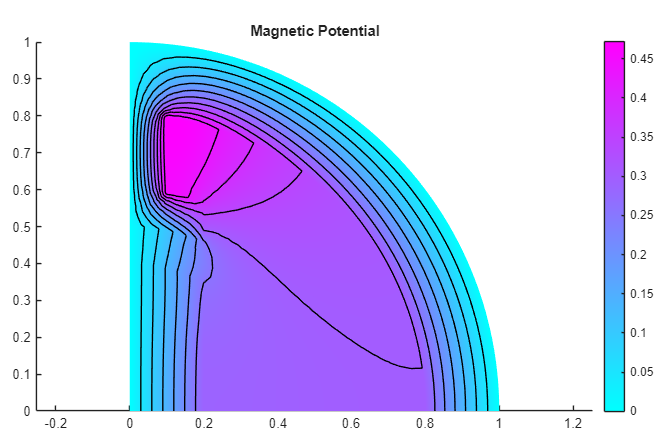Add the magnetic field data to the plot. Use the `FaceAlpha` parameter to make the quiver plot for magnetic field more visible.

```figure pdeplot(emagmodel,"XYData",R.MagneticPotential, ... "FlowData",[R.MagneticField.Hx, ... R.MagneticField.Hy], ... "Contour","on", ... "FaceAlpha",0.5) title "Magnetic Potential and Field"```